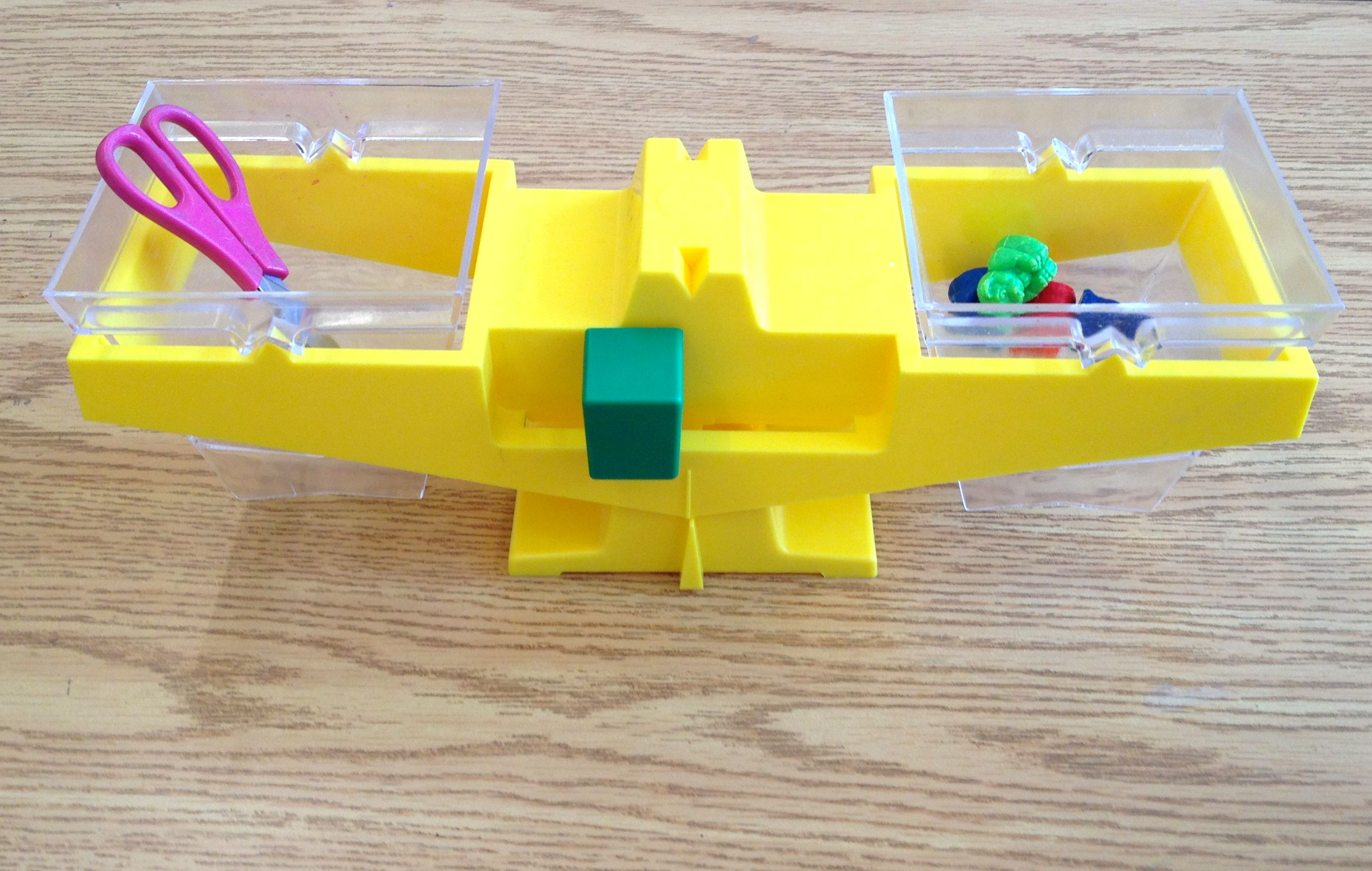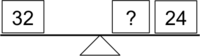# How Heavy?

Alignments to Content Standards: 3.MD.A.2Students will need various items, a balance scale, a large set of cubes such as unifix or snap cubes, and a recording sheet. They choose an item to measure. Using the balance scale, they put the item on one side of the balance scale. Then they put enough cubes on the other side of the scale to make it balance. They remove the cubes, count them, and record the result. For example, if a small book balances with 12 cubes, they should write, "The book has the same mass as 12 cubes." They continue same routine three more times with different items.

## IM Commentary

The purpose of this task is for students to measure the mass of objects with a balance scale. It would be worthwhile measuring the mass of the same object with a different units as well so that students understand that if you use a smaller unit, you will need more of them to equal the same mass. Students should follow up this measuring activity with finding the mass of an object with a scale that gives the mass as a numeric value, such as a kitchen scale. At some point, students should measure the mass of objects that are close to 1 gram or 1 kilogram so they can develop intuition for these units, and they should understand that if a book has a mass of approximately 1 kg, then it will take about 30 books to balance with a 30-kilogram child on an appropriately sized balance scale. Since the balance scale is not precise enough to measure objects that are close to 1 gram and might not fit objects that are approximately 1 kg in mass, other tools would be needed to do this.

It is common for teachers and curriculum writers to use a balance scale as a metaphor for an equation; for example, they might be asked to write an equation for a balance scale like this:Note that without an experience of actually using a balance scale, this representation is almost as abstract as an equation like 32 = ? + 24 is. Moreover, the standard representations of a balance scale such as the one shown above disregard the concept of a moment-arm, which takes into account not just the mass of an object, but its distance from the balance point. Finally, the concept of mass itself is more abstract than many people realize, in part because it is measured indirectly through weight. In summary, using a balance scale to represent an equation should be used cautiously and only once students have a solid understanding of the concept of mass.

## Solution

The scissors have the same mass as 8 marbles.

The hacky sack has the same mass as 20 marbles.

The eraser has the same mass as 2 marbles.

The racquet ball has the same mass as 9 marbles.